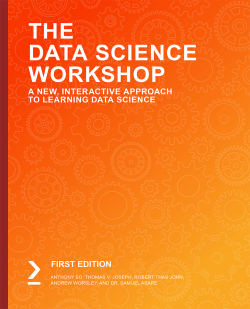# The Data Science Workshop

By Anthony So , Thomas V. Joseph , Robert Thas John and 2 more
• \$41.99 Print
• \$28.99 eBook
• \$12.99 eBook + Subscription
What do you get with a Packt Subscription?

• Constantly updated with 100+ new titles each month
• Breadth and depth in over 1,000+ technologies

You already know you want to learn data science, and a smarter way to learn data science is to learn by doing. The Data Science Workshop focuses on building up your practical skills so that you can understand how to develop simple machine learning models in Python or even build an advanced model for detecting potential bank frauds with effective modern data science. You'll learn from real examples that lead to real results.

Throughout The Data Science Workshop, you'll take an engaging step-by-step approach to understanding data science. You won't have to sit through any unnecessary theory. If you're short on time you can jump into a single exercise each day or spend an entire weekend training a model using sci-kit learn. It's your choice. Learning on your terms, you'll build up and reinforce key skills in a way that feels rewarding.

Every physical print copy of The Data Science Workshop unlocks access to the interactive edition. With videos detailing all exercises and activities, you'll always have a guided solution. You can also benchmark yourself against assessments, track progress, and receive content updates. You'll even earn a secure credential that you can share and verify online upon completion. It's a premium learning experience that's included with your printed copy. To redeem, follow the instructions located at the start of your data science book.

Fast-paced and direct, The Data Science Workshop is the ideal companion for data science beginners. You'll learn about machine learning algorithms like a data scientist, learning along the way. This process means that you'll find that your new skills stick, embedded as best practice. A solid foundation for the years ahead.

Publication date:
January 2020
Publisher
Packt
Pages
818
ISBN
9781838981266

# 1. Introduction to Data Science in Python

Overview

By the end of this chapter, you will be able to explain what data science is and distinguish between supervised and unsupervised learning. You will also be able to explain what machine learning is and distinguish between regression, classification, and clustering problems. You will be able to create and manipulate different types of Python variable, including core variables, lists, and dictionaries. You will build a `for` loop, print results using f-strings, and define functions. You will also import Python packages and load data in different formats using `pandas`. You will also get your first taste of training a model using scikit-learn.

This very first chapter will introduce you to the field of data science and walk you through an overview of Python's core concepts and their application in the world of data science.

# Introduction

Welcome to the fascinating world of data science! We are sure you must be pretty excited to start your journey and learn interesting and exciting techniques and algorithms. This is exactly what this book is intended for.

But before diving into it, let's define what data science is: it is a combination of multiple disciplines, including business, statistics, and programming, that intends to extract meaningful insights from data by running controlled experiments similar to scientific research.

The objective of any data science project is to derive valuable knowledge for the business from data in order to make better decisions. It is the responsibility of data scientists to define the goals to be achieved for a project. This requires business knowledge and expertise. In this book, you will be exposed to some examples of data science tasks from real-world datasets.

Statistics is a mathematical field used for analyzing and finding patterns from data. A lot of the newest and most advanced techniques still rely on core statistical approaches. This book will present to you the basic techniques required to understand the concepts we will be covering.

With an exponential increase in data generation, more computational power is required for processing it efficiently. This is the reason why programming is a required skill for data scientists. You may wonder why we chose Python for this Workshop. That's because Python is one of the most popular programming languages for data science. It is extremely easy to learn how to code in Python thanks to its simple and easily readable syntax. It also has an incredible number of packages available to anyone for free, such as pandas, scikit-learn, TensorFlow, and PyTorch. Its community is expanding at an incredible rate, adding more and more new functionalities and improving its performance and reliability. It's no wonder companies such as Facebook, Airbnb, and Google are using it as one of their main stacks. No prior knowledge of Python is required for this book. If you do have some experience with Python or other programming languages, then this will be an advantage, but all concepts will be fully explained, so don't worry if you are new to programming.

# Application of Data Science

As mentioned in the introduction, data science is a multidisciplinary approach to analyzing and identifying complex patterns and extracting valuable insights from data. Running a data science project usually involves multiple steps, including the following:

1. Defining the business problem to be solved
2. Collecting or extracting existing data
3. Analyzing, visualizing, and preparing data
4. Training a model to spot patterns in data and make predictions
5. Assessing a model's performance and making improvements
6. Communicating and presenting findings and gained insights
7. Deploying and maintaining a model

As its name implies, data science projects require data, but it is actually more important to have defined a clear business problem to solve first. If it's not framed correctly, a project may lead to incorrect results as you may have used the wrong information, not prepared the data properly, or led a model to learn the wrong patterns. So, it is absolutely critical to properly define the scope and objective of a data science project with your stakeholders.

There are a lot of data science applications in real-world situations or in business environments. For example, healthcare providers may train a model for predicting a medical outcome or its severity based on medical measurements, or a high school may want to predict which students are at risk of dropping out within a year's time based on their historical grades and past behaviors. Corporations may be interested to know the likelihood of a customer buying a certain product based on his or her past purchases. They may also need to better understand which customers are more likely to stop using existing services and churn. These are examples where data science can be used to achieve a clearly defined goal, such as increasing the number of patients detected with a heart condition at an early stage or reducing the number of customers canceling their subscriptions after six months. That sounds exciting, right? Soon enough, you will be working on such interesting projects.

## What Is Machine Learning?

When we mention data science, we usually think about machine learning, and some people may not understand the difference between them. Machine learning is the field of building algorithms that can learn patterns by themselves without being programmed explicitly. So machine learning is a family of techniques that can be used at the modeling stage of a data science project.

Machine learning is composed of three different types of learning:

• Supervised learning
• Unsupervised learning
• Reinforcement learning

### Supervised Learning

Supervised learning refers to a type of task where an algorithm is trained to learn patterns based on prior knowledge. That means this kind of learning requires the labeling of the outcome (also called the response variable, dependent variable, or target variable) to be predicted beforehand. For instance, if you want to train a model that will predict whether a customer will cancel their subscription, you will need a dataset with a column (or variable) that already contains the churn outcome (cancel or not cancel) for past or existing customers. This outcome has to be labeled by someone prior to the training of a model. If this dataset contains 5,000 observations, then all of them need to have the outcome being populated. The objective of the model is to learn the relationship between this outcome column and the other features (also called independent variables or predictor variables). Following is an example of such a dataset: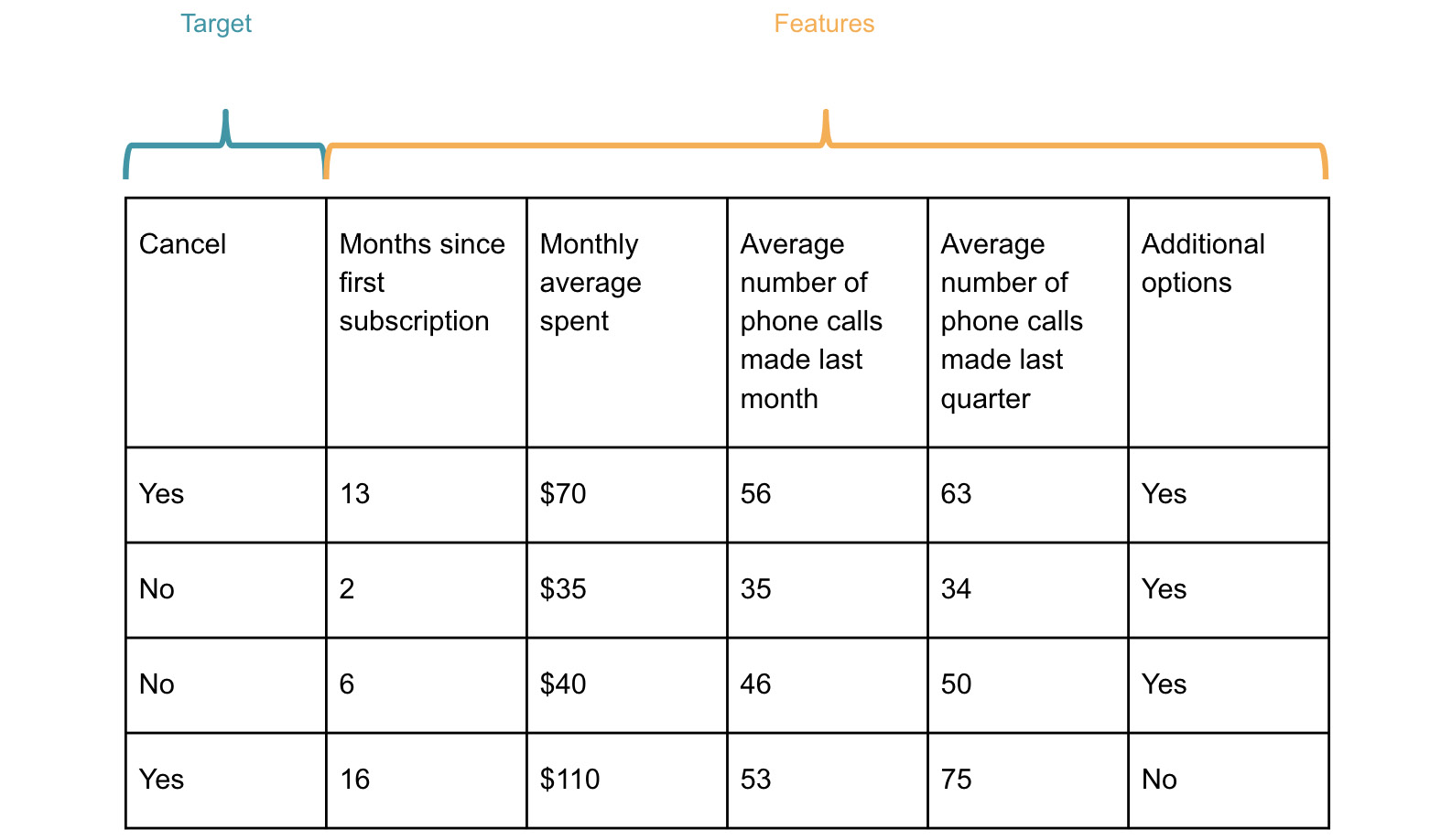Figure 1.1: Example of customer churn dataset

The `Cancel` column is the response variable. This is the column you are interested in, and you want the model to predict accurately the outcome for new input data (in this case, new customers). All the other columns are the predictor variables.

The model, after being trained, may find the following pattern: a customer is more likely to cancel their subscription after 12 months and if their average monthly spent is over `\$50`. So, if a new customer has gone through 15 months of subscription and is spending \$85 per month, the model will predict this customer will cancel their contract in the future.

When the response variable contains a limited number of possible values (or classes), it is a classification problem (you will learn more about this in Chapter 3, Binary Classification, and Chapter 4, Multiclass Classification with RandomForest). The model will learn how to predict the right class given the values of the independent variables. The churn example we just mentioned is a classification problem as the response variable can only take two different values: `yes` or `no`.

On the other hand, if the response variable can have a value from an infinite number of possibilities, it is called a regression problem.

An example of a regression problem is where you are trying to predict the exact number of mobile phones produced every day for some manufacturing plants. This value can potentially range from 0 to an infinite number (or a number big enough to have a large range of potential values), as shown in Figure 1.2.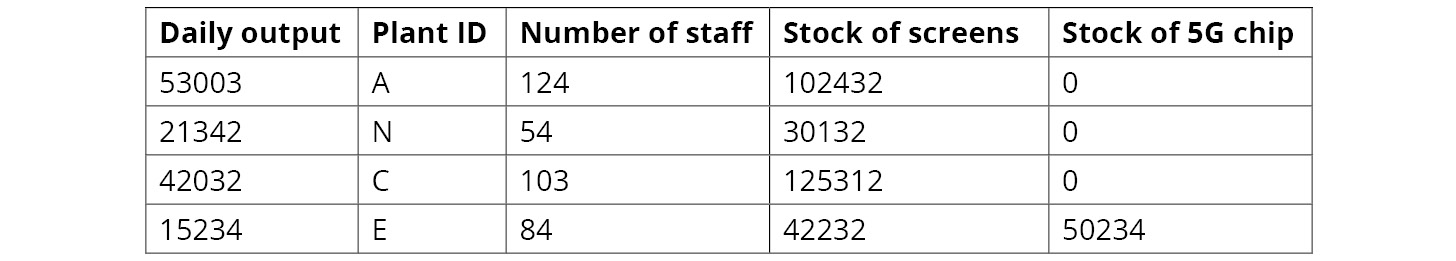Figure 1.2: Example of a mobile phone production dataset

In the preceding figure, you can see that the values for `Daily output` can take any value from `15000` to more than `50000`. This is a regression problem, which we will look at in Chapter 2, Regression.

### Unsupervised Learning

Unsupervised learning is a type of algorithm that doesn't require any response variables at all. In this case, the model will learn patterns from the data by itself. You may ask what kind of pattern it can find if there is no target specified beforehand.

This type of algorithm usually can detect similarities between variables or records, so it will try to group those that are very close to each other. This kind of algorithm can be used for clustering (grouping records) or dimensionality reduction (reducing the number of variables). Clustering is very popular for performing customer segmentation, where the algorithm will look to group customers with similar behaviors together from the data. Chapter 5, Performing Your First Cluster Analysis, will walk you through an example of clustering analysis.

### Reinforcement Learning

Reinforcement learning is another type of algorithm that learns how to act in a specific environment based on the feedback it receives. You may have seen some videos where algorithms are trained to play Atari games by themselves. Reinforcement learning techniques are being used to teach the agent how to act in the game based on the rewards or penalties it receives from the game.

For instance, in the game Pong, the agent will learn to not let the ball drop after multiple rounds of training in which it receives high penalties every time the ball drops.

Note

Reinforcement learning algorithms are out of scope and will not be covered in this book.

# Overview of Python

As mentioned earlier, Python is one of the most popular programming languages for data science. But before diving into Python's data science applications, let's have a quick introduction to some core Python concepts.

## Types of Variable

In Python, you can handle and manipulate different types of variables. Each has its own specificities and benefits. We will not go through every single one of them but rather focus on the main ones that you will have to use in this book. For each of the following code examples, you can run the code in Google Colab to view the given output.

### Numeric Variables

The most basic variable type is numeric. This can contain integer or decimal (or float) numbers, and some mathematical operations can be performed on top of them.

Let's use an integer variable called `var1` that will take the value `8` and another one called `var2` with the value `160.88`, and add them together with the `+` operator, as shown here:

```var1 = 8
var2 = 160.88
var1 + var2```

You should get the following output:Figure 1.3: Output of the addition of two variables

In Python, you can perform other mathematical operations on numerical variables, such as multiplication (with the `*` operator) and division (with `/`).

### Text Variables

Another interesting type of variable is `string`, which contains textual information. You can create a variable with some specific text using the single or double quote, as shown in the following example:

```var3 = 'Hello, '
var4 = 'World'```

In order to display the content of a variable, you can call the `print()` function:

```print(var3)
print(var4)```

You should get the following output:Figure 1.4: Printing the two text variables

Python also provides an interface called f-strings for printing text with the value of defined variables. It is very handy when you want to print results with additional text to make it more readable and interpret results. It is also quite common to use f-strings to print logs. You will need to add `f` before the quotes (or double quotes) to specify that the text will be an f-string. Then you can add an existing variable inside the quotes and display the text with the value of this variable. You need to wrap the variable with curly brackets, `{}`. For instance, if we want to print `Text:` before the values of `var3` and `var4`, we will write the following code:

`print(f"Text: {var3} {var4}!")`

You should get the following output: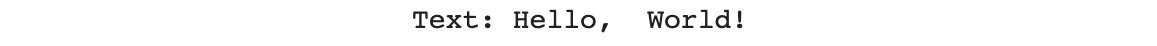Figure 1.5: Printing with f-strings

You can also perform some text-related transformations with string variables, such as capitalizing or replacing characters. For instance, you can concatenate the two variables together with the `+` operator:

`var3 + var4`

You should get the following output: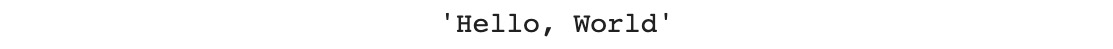Figure 1.6: Concatenation of the two text variables

### Python List

Another very useful type of variable is the list. It is a collection of items that can be changed (you can add, update, or remove items). To declare a list, you will need to use square brackets, `[]`, like this:

```var5 = ['I', 'love', 'data', 'science']
print(var5)```

You should get the following output: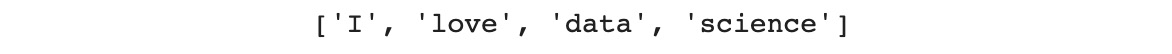Figure 1.7: List containing only string items

A list can have different item types, so you can mix numerical and text variables in it:

```var6 = ['Packt', 15019, 2020, 'Data Science']
print(var6)```

You should get the following output: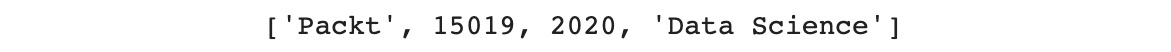Figure 1.8: List containing numeric and string items

An item in a list can be accessed by its index (its position in the list). To access the first (index 0) and third elements (index 2) of a list, you do the following:

```print(var6)
print(var6)```

Note

In Python, all indexes start at `0`.

You should get the following output:Figure 1.9: The first and third items in the var6 list

Python provides an API to access a range of items using the `:` operator. You just need to specify the starting index on the left side of the operator and the ending index on the right side. The ending index is always excluded from the range. So, if you want to get the first three items (index 0 to 2), you should do as follows:

`print(var6[0:3])`

You should get the following output: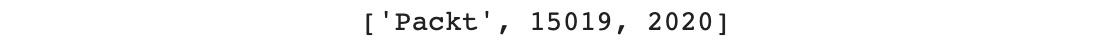Figure 1.10: The first three items of var6

You can also iterate through every item of a list using a `for` loop. If you want to print every item of the `var6` list, you should do this:

```for item in var6:
print(item)```

You should get the following output:Figure 1.11: Output of the for loop

You can add an item at the end of the list using the `.append()` method:

```var6.append('Python')
print(var6)```

You should get the following output:Figure 1.12: Output of var6 after inserting the 'Python' item

To delete an item from the list, you use the `.remove()` method:

```var6.remove(15019)
print(var6)```

You should get the following output: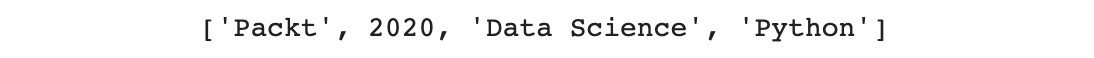Figure 1.13: Output of var6 after removing the '15019' item

### Python Dictionary

Another very popular Python variable used by data scientists is the dictionary type. For example, it can be used to load JSON data into Python so that it can then be converted into a DataFrame (you will learn more about the JSON format and DataFrames in the following sections). A dictionary contains multiple elements, like a list, but each element is organized as a key-value pair. A dictionary is not indexed by numbers but by keys. So, to access a specific value, you will have to call the item by its corresponding key. To define a dictionary in Python, you will use curly brackets, `{}`, and specify the keys and values separated by `:`, as shown here:

```var7 = {'Topic': 'Data Science', 'Language': 'Python'}
print(var7)```

You should get the following output: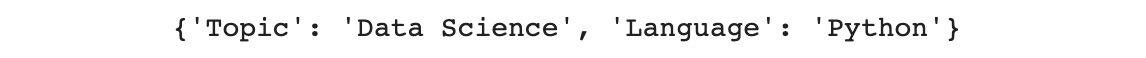Figure 1.14: Output of var7

To access a specific value, you need to provide the corresponding key name. For instance, if you want to get the value `Python`, you do this:

`var7['Language']`

You should get the following output:Figure 1.15: Value for the 'Language' key

Note

Each key-value pair in a dictionary needs to be unique.

Python provides a method to access all the key names from a dictionary, `.keys()`, which is used as shown in the following code snippet:

`var7.keys()`

You should get the following output:Figure 1.16: List of key names

There is also a method called `.values()`, which is used to access all the values of a dictionary:

`var7.values()`

You should get the following output: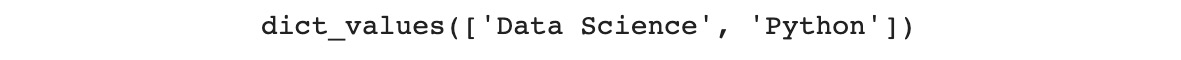Figure 1.17: List of values

You can iterate through all items from a dictionary using a `for` loop and the `.items()` method, as shown in the following code snippet:

```for key, value in var7.items():
print(key)
print(value)```

You should get the following output:Figure 1.18: Output after iterating through the items of a dictionary

You can add a new element in a dictionary by providing the key name like this:

```var7['Publisher'] = 'Packt'
print(var7)```

You should get the following output: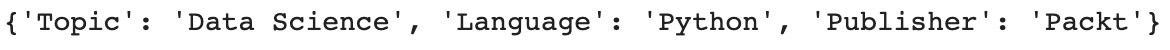Figure 1.19: Output of a dictionary after adding an item

You can delete an item from a dictionary with the `del` command:

```del var7['Publisher']
print(var7)```

You should get the following output: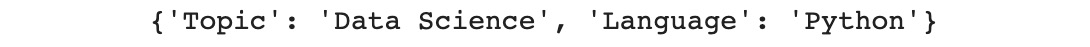Figure 1.20: Output of a dictionary after removing an item

In Exercise 1.01, we will be looking to use these concepts that we've just looked at.

Note

If you are interested in exploring Python in more depth, head over to our website (https://packt.live/2FcXpOp) to get yourself the Python Workshop.

## Exercise 1.01: Creating a Dictionary That Will Contain Machine Learning Algorithms

In this exercise, we will create a dictionary using Python that will contain a collection of different machine learning algorithms that will be covered in this book.

Note

Every exercise and activity in this book is to be executed on Google Colab.

1. Open on a new Colab notebook.
2. Create a list called `algorithm` that will contain the following elements: `Linear Regression`, `Logistic Regression`, `RandomForest`, and `a3c`:
`algorithm = ['Linear Regression', 'Logistic Regression', 'RandomForest', 'a3c']`
3. Now, create a list called `learning` that will contain the following elements: `Supervised`, `Supervised`, `Supervised`, and `Reinforcement`:
`learning = ['Supervised', 'Supervised', 'Supervised', 'Reinforcement']`
4. Create a list called `algorithm_type` that will contain the following elements: `Regression`, `Classification`, `Regression` or `Classification`, and `Game AI`:
`algorithm_type = ['Regression', 'Classification', 'Regression or Classification', 'Game AI']`
5. Add an item called `k-means` into the `algorithm` list using the `.append()` method:
`algorithm.append('k-means')`
6. Display the content of `algorithm` using the `print()` function:
`print(algorithm)`

You should get the following output: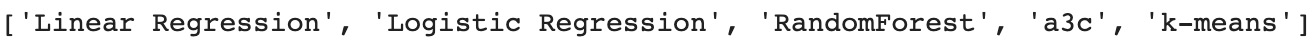Figure 1.21: Output of 'algorithm'

From the preceding output, we can see that we added the `k-means` item to the list.

7. Now, add the `Unsupervised` item into the `learning` list using the `.append()` method:
`learning.append('Unsupervised')`
8. Display the content of `learning` using the `print()` function:
`print(learning)`

You should get the following output: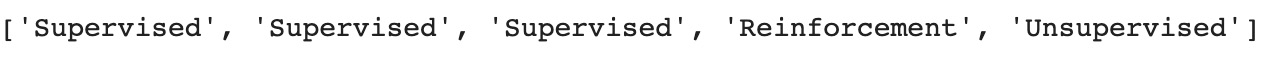Figure 1.22: Output of 'learning'

From the preceding output, we can see that we added the `Unsupervised` item into the list.

9. Add the `Clustering` item into the `algorithm_type` list using the `.append()` method:
`algorithm_type.append('Clustering')`
10. Display the content of `algorithm_type` using the `print()` function:
`print(algorithm_type)`

You should get the following output: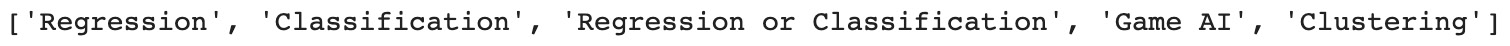Figure 1.23: Output of 'algorithm_type'

From the preceding output, we can see that we added the `Clustering` item into the list.

11. Create an empty dictionary called `machine_learning` using curly brackets, `{}`:
`machine_learning = {}`
12. Create a new item in `machine_learning` with the key as `algorithm` and the value as all the items from the `algorithm` list:
`machine_learning['algorithm'] = algorithm`
13. Display the content of `machine_learning` using the `print()` function.
`print(machine_learning)`

You should get the following output: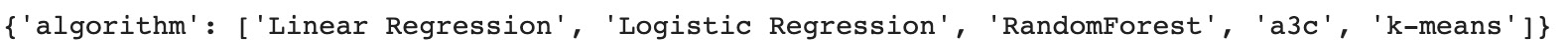Figure 1.24: Output of 'machine_learning'

From the preceding output, we notice that we have created a dictionary from the `algorithm` list.

14. Create a new item in `machine_learning` with the key as `learning` and the value as all the items from the `learning` list:
`machine_learning['learning'] = learning`
15. Now, create a new item in `machine_learning` with the key as `algorithm_type` and the value as all the items from the algorithm_type list:
`machine_learning['algorithm_type'] = algorithm_type`
16. Display the content of `machine_learning` using the `print()` function.
`print(machine_learning)`

You should get the following output: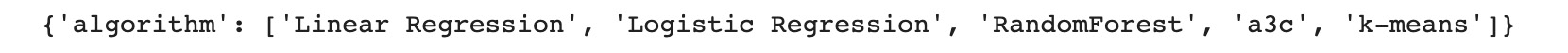Figure 1.25: Output of 'machine_learning'

17. Remove the `a3c` item from the `algorithm` key using the `.remove()` method:
`machine_learning['algorithm'].remove('a3c')`
18. Display the content of the `algorithm` item from the `machine_learning` dictionary using the `print()` function:
`print(machine_learning['algorithm'])`

You should get the following output: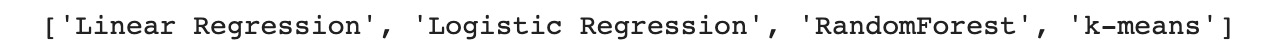Figure 1.26: Output of 'algorithm' from 'machine_learning'

19. Remove the `Reinforcement` item from the `learning` key using the `.remove()` method:
`machine_learning['learning'].remove('Reinforcement')`
20. Remove the `Game AI` item from the `algorithm_type` key using the `.remove()` method:
`machine_learning['algorithm_type'].remove('Game AI')`
21. Display the content of `machine_learning` using the `print()` function:
`print(machine_learning)`

You should get the following output: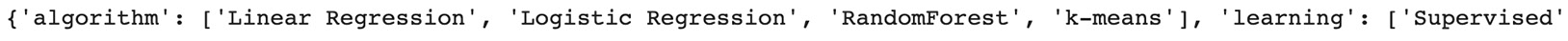Figure 1.27: Output of 'machine_learning'

You have successfully created a dictionary containing the machine learning algorithms that you will come across in this book. You learned how to create and manipulate Python lists and dictionaries.

In the next section, you will learn more about the two main Python packages used for data science:

• `pandas`
• `scikit-learn`

# Python for Data Science

Python offers an incredible number of packages for data science. A package is a collection of prebuilt functions and classes shared publicly by its author(s). These packages extend the core functionalities of Python. The Python Package Index (https://packt.live/37iTRXc) lists all the packages available in Python.

In this section, we will present to you two of the most popular ones: `pandas` and `scikit-learn`.

## The pandas Package

The pandas package provides an incredible amount of APIs for manipulating data structures. The two main data structures defined in the `pandas` package are `DataFrame` and `Series`.

### DataFrame and Series

A `DataFrame` is a tabular data structure that is represented as a two-dimensional table. It is composed of rows, columns, indexes, and cells. It is very similar to a sheet in Excel or a table in a database: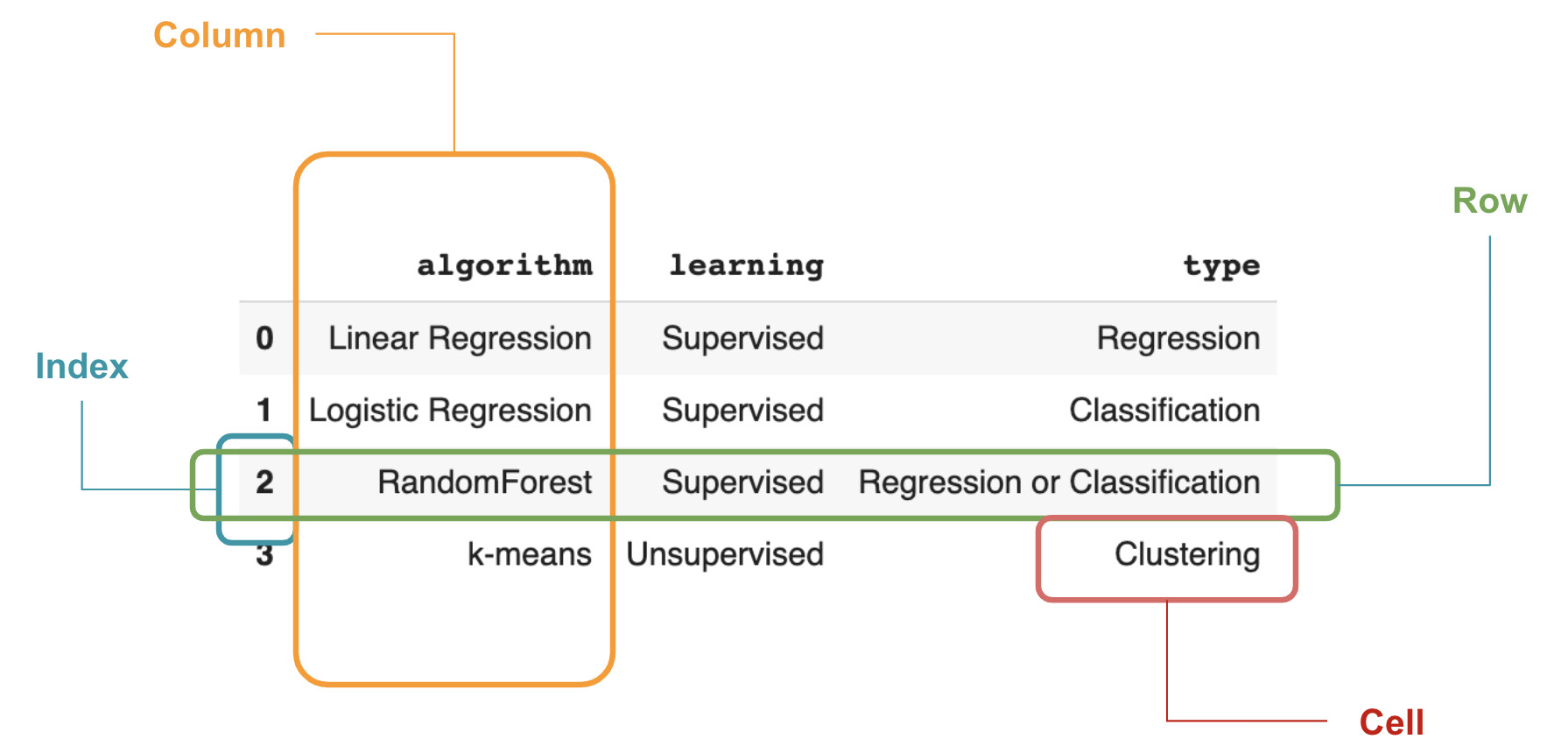Figure 1.28: Components of a DataFrame

In Figure 1.28, there are three different columns: `algorithm`, `learning`, and `type`. Each of these columns (also called variables) contains a specific type of information. For instance, the `algorithm` variable lists the names of different machine learning algorithms.

A row stores the information related to a record (also called an observation). For instance, row number `2` (index number `2`) refers to the `RandomForest` record and all its attributes are stored in the different columns.

Finally, a cell is the value of a given row and column. For example, `Clustering` is the value of the cell of the row index `2` and the `type` column. You can see it as the intersection of a specified row and column.

So, a DataFrame is a structured representation of some data organized by rows and columns. A row represents an observation and each column contains the value of its attributes. This is the most common data structure used in data science.

In pandas, a DataFrame is represented by the `DataFrame` class. A `pandas` DataFrame is composed of `pandas` Series, which are 1-dimensional arrays. A `pandas` Series is basically a single column in a DataFrame.

Data is usually classified into two groups: structured and unstructured. Think of structured data as database tables or Excel spreadsheets where each column and row has a predefined structure. For example, in a table or spreadsheet that lists all the employees of a company, every record will follow the same pattern, such as the first column containing the date of birth, the second and third ones being for first and last names, and so on.

On the other hand, unstructured data is not organized with predefined and static patterns. Text and images are good examples of unstructured data. If you read a book and look at each sentence, it will not be possible for you to say that the second word of a sentence is always a verb or a person's name; it can be anything depending on how the author wanted to convey the information they wanted to share. Each sentence has its own structure and will be different from the last. Similarly, for a group of images, you can't say that pixels 20 to 30 will always represent the eye of a person or the wheel of a car: it will be different for each image.

Data can come from different data sources: there could be flat files, data storage, or Application Programming Interface (API) feeds, for example. In this book, we will work with flat files such as CSVs, Excel spreadsheets, or JSON. All these types of files are storing information with their own format and structure.

We'll have a look at the CSV file first.

### CSV Files

CSV files use the comma character—`,`—to separate columns and newlines for a new row. The previous example of a DataFrame would look like this in a CSV file:

```algorithm,learning,type
Linear Regression,Supervised,Regression
Logistic Regression,Supervised,Classification
RandomForest,Supervised,Regression or Classification
k-means,Unsupervised,Clustering```

In Python, you need to first import the packages you require before being able to use them. To do so, you will have to use the `import` command. You can create an alias of each imported package using the `as` keyword. It is quite common to import the `pandas` package with the alias `pd`:

`import pandas as pd`

pandas provides a `.read_csv()` method to easily load a CSV file directly into a DataFrame. You just need to provide the path or the URL to the CSV file:

`pd.read_csv('https://raw.githubusercontent.com/PacktWorkshops/The-Data-Science-Workshop/master/Chapter01/Dataset/csv_example.csv')`

You should get the following output: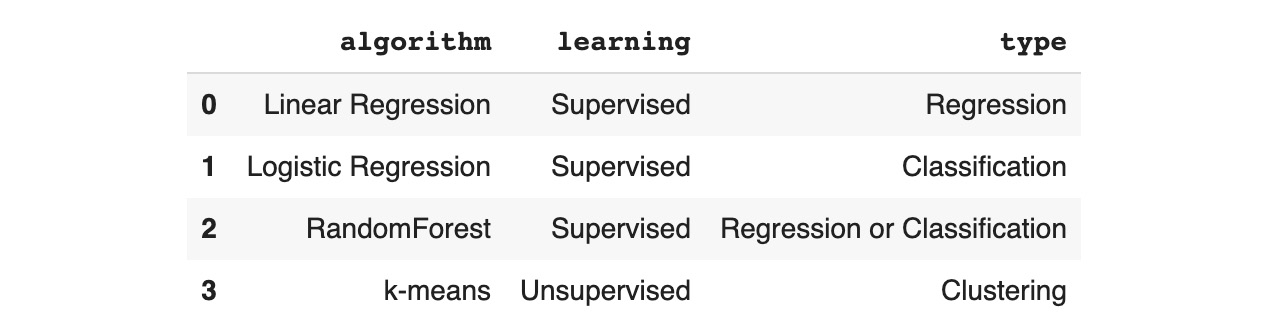Note

In this book, we will be loading datasets stored in the Packt GitHub repository: https://packt.live/2ucwsId.

GitHub wraps stored data into its own specific format. To load the original version of a dataset, you will need to load the raw version of it by clicking on the Raw button and copying the URL provided on your browser.

Have a look at Figure 1.30: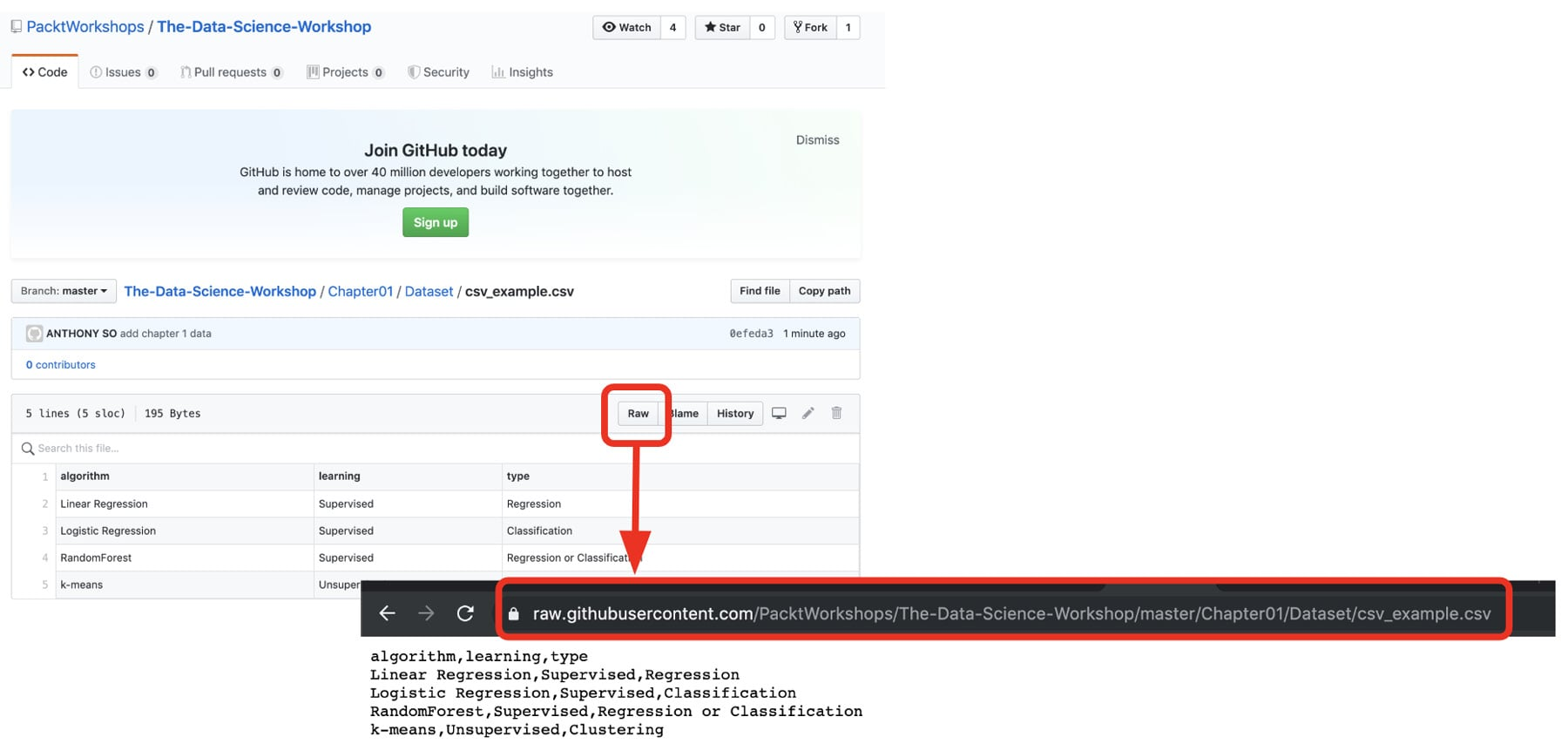Figure 1.30: Getting the URL of a raw dataset on GitHub

Excel is a Microsoft tool and is very popular in the industry. It has its own internal structure for recording additional information, such as the data type of each cell or even Excel formulas. There is a specific method in pandas to load Excel spreadsheets called `.read_excel()`:

`pd.read_excel('https://github.com/PacktWorkshops/The-Data-Science-Workshop/blob/master/Chapter01/Dataset/excel_example.xlsx?raw=true')`

You should get the following output: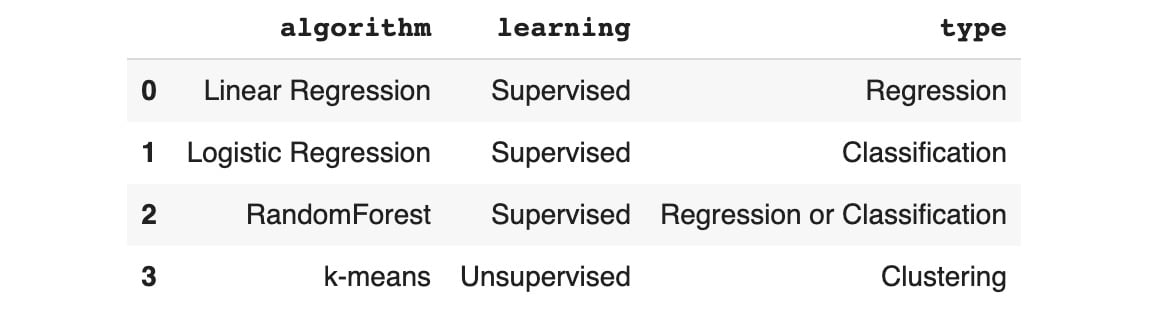### JSON

JSON is a very popular file format, mainly used for transferring data from web APIs. Its structure is very similar to that of a Python dictionary with key-value pairs. The example DataFrame we used before would look like this in JSON format:

```{
"algorithm":{
"0":"Linear Regression",
"1":"Logistic Regression",
"2":"RandomForest",
"3":"k-means"
},
"learning":{
"0":"Supervised",
"1":"Supervised",
"2":"Supervised",
"3":"Unsupervised"
},
"type":{
"0":"Regression",
"1":"Classification",
"2":"Regression or Classification",
"3":"Clustering"
}
}```

As you may have guessed, there is a `pandas` method for reading JSON data as well, and it is called `.read_json()`:

`pd.read_json('https://raw.githubusercontent.com/PacktWorkshops/The-Data-Science-Workshop/master/Chapter01/Dataset/json_example.json')`

You should get the following output: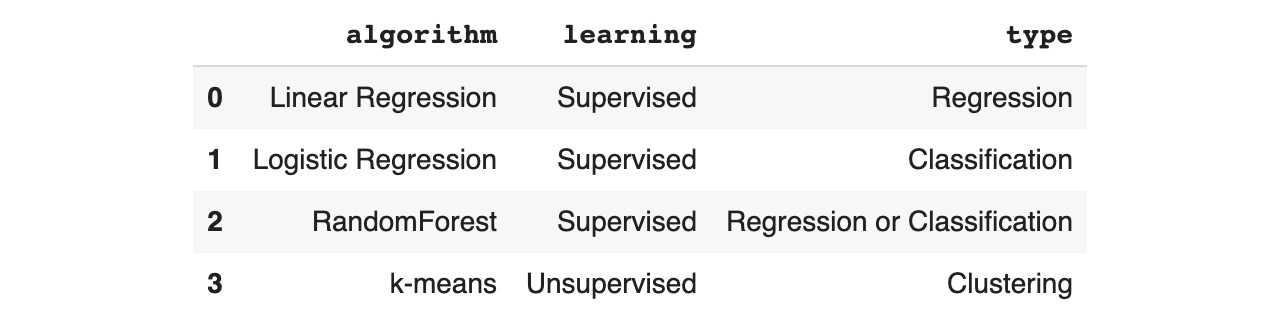`pandas` provides more methods to load other types of files. The full list can be found in the following documentation: https://packt.live/2FiYB2O.

pandas is not limited to only loading data into DataFrames; it also provides a lot of other APIs for creating, analyzing, or transforming DataFrames. You will be introduced to some of its most useful methods in the following chapters.

## Exercise 1.02: Loading Data of Different Formats into a pandas DataFrame

In this exercise, we will practice loading different data formats, such as CSV, TSV, and XLSX, into pandas DataFrames. The dataset we will use is the Top 10 Postcodes for the First Home Owner Grants dataset (this is a grant provided by the Australian government to help first-time real estate buyers). It lists the 10 postcodes (also known as zip codes) with the highest number of First Home Owner grants.

In this dataset, you will find the number of First Home Owner grant applications for each Australian postcode and the corresponding suburb.

Note

This dataset can be found on our GitHub repository at https://packt.live/2FgAT7d.

Also, it is publicly available here: https://packt.live/2ZJBYhi.

1. Open a new Colab notebook.
2. Import the pandas package, as shown in the following code snippet:
`import pandas as pd`
3. Create a new variable called `csv_url` containing the URL to the raw CSV file:
`csv_url = 'https://raw.githubusercontent.com/PacktWorkshops/The-Data-Science-Workshop/master/Chapter01/Dataset/overall_topten_2012-2013.csv'`
4. Load the CSV file into a DataFrame using the pandas `.read_csv()` method. The first row of this CSV file contains the name of the file, as you can see in the following screenshot. You will need to exclude it by using the `skiprows=1` parameter. Save the result in a variable called `csv_df` and print it:
```csv_df = pd.read_csv(csv_url, skiprows=1)
csv_df```

You should get the following output: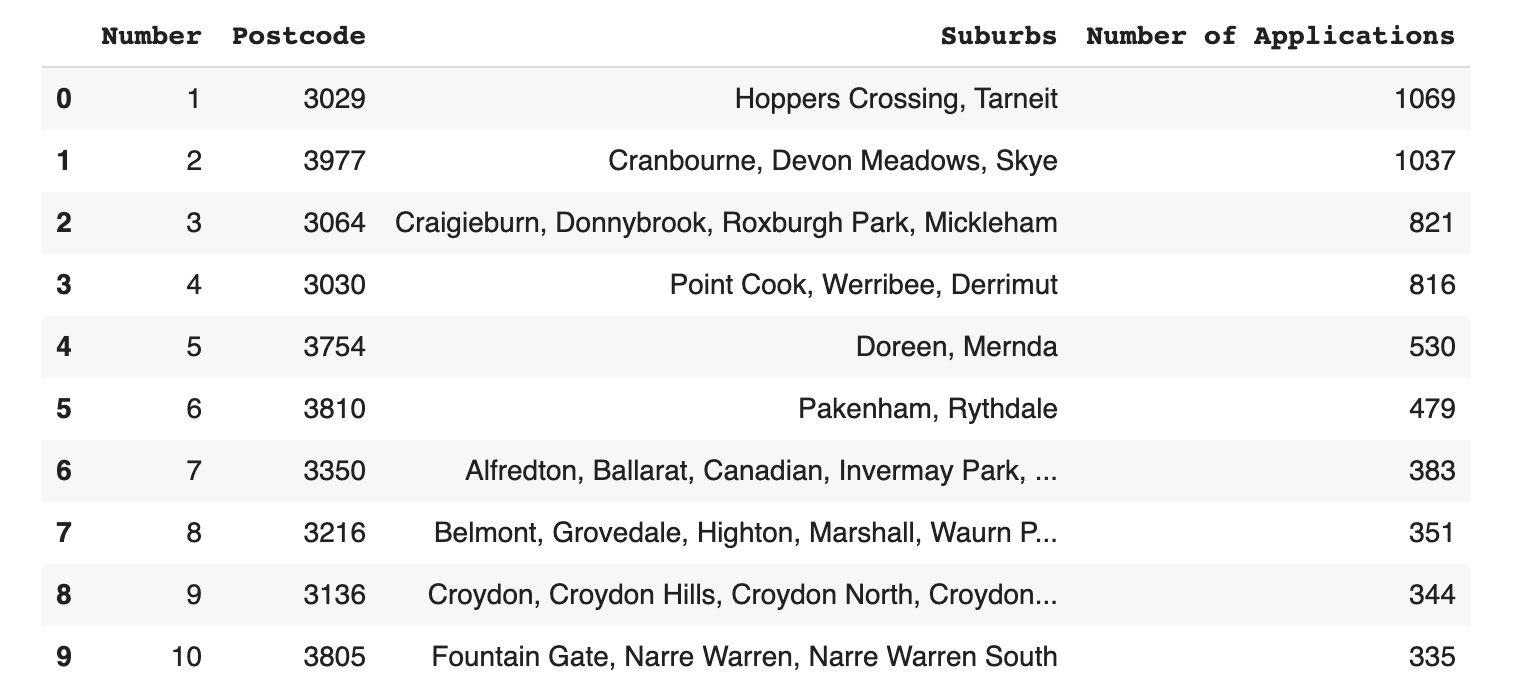5. Create a new variable called `tsv_url` containing the URL to the raw TSV file:
`tsv_url = 'https://raw.githubusercontent.com/PacktWorkshops/The-Data-Science-Workshop/master/Chapter01/Dataset/overall_topten_2012-2013.tsv'`

Note

A TSV file is similar to a CSV file but instead of using the comma character (`,`) as a separator, it uses the tab character (`\t`).

6. Load the TSV file into a DataFrame using the pandas .`read_csv()` method and specify the `skiprows=1` and `sep='\t'` parameters. Save the result in a variable called `tsv_df` and print it:
```tsv_df = pd.read_csv(tsv_url, skiprows=1, sep='\t')
tsv_df```

You should get the following output:7. Create a new variable called `xlsx_url` containing the URL to the raw Excel spreadsheet:
`xlsx_url = 'https://github.com/PacktWorkshops/The-Data-Science-Workshop/blob/master/Chapter01/Dataset/overall_topten_2012-2013.xlsx?raw=true'`
8. Load the Excel spreadsheet into a DataFrame using the pandas `.read_excel()` method. Save the result in a variable called `xlsx_df` and print it:
```xlsx_df = pd.read_excel(xlsx_url)
xlsx_df```

You should get the following output: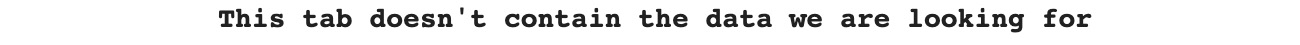By default, `.read_excel()` loads the first sheet of an Excel spreadsheet. In this example, the data is actually stored in the second sheet.

9. Load the Excel spreadsheet into a Dataframe using the pandas `.read_excel()` method and specify the `skiprows=1` and `sheetname=1` parameters. Save the result in a variable called `xlsx_df1` and print it:
```xlsx_df1 = pd.read_excel(xlsx_url, skiprows=1, sheet_name=1)
xlsx_df1```

You should get the following output: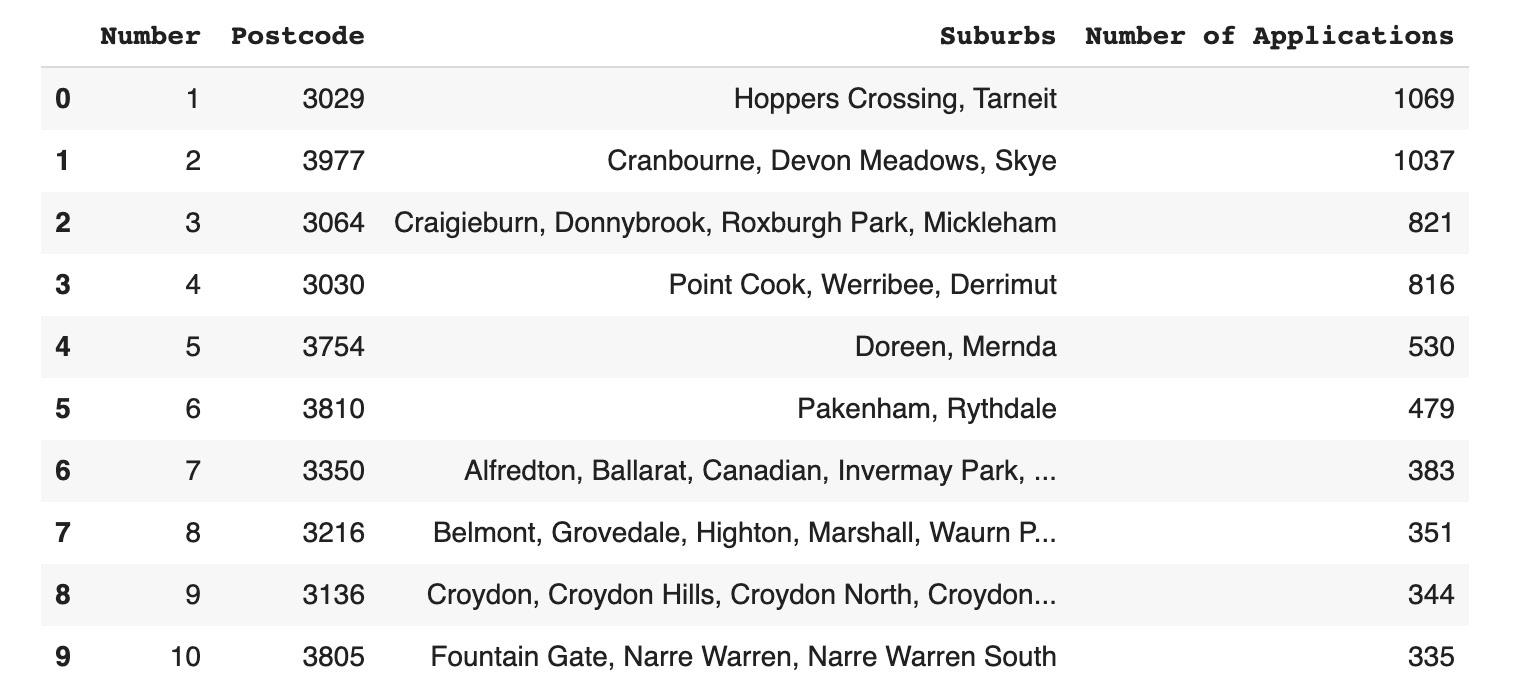In this exercise, we learned how to load the Top 10 Postcodes for First Home Buyer Grants dataset from different file formats.

In the next section, we will be introduced to scikit-learn.

# Scikit-Learn

Scikit-learn (also referred to as `sklearn`) is another extremely popular package used by data scientists. The main purpose of `sklearn` is to provide APIs for processing data and training machine learning algorithms. But before moving ahead, we need to know what a model is.

### What Is a Model?

A machine learning model learns patterns from data and creates a mathematical function to generate predictions. A supervised learning algorithm will try to find the relationship between a response variable and the given features.

Have a look at the following example.

A mathematical function can be represented as a function, ƒ(), that is applied to some input variables, X (which is composed of multiple features), and will calculate an output (or prediction), ŷ:Figure 1.37: Function f(X)

The function, ƒ(), can be quite complex and have different numbers of parameters. If we take a linear regression (this will be presented in more detail in Chapter 2, Regression) as an example, the model parameters can be represented as W=( w1, w2, ... , wn). So, the function we saw earlier will become as follows:Figure 1.38: Function for linear regression

A machine learning algorithm will receive some examples of input X with the relevant output, y, and its goal will be to find the values of ( w1, w2, ... , wn) that will minimize the difference between its prediction, ŷ and the true output, y.

The previous formulas can be a bit intimidating, but this is actually quite simple. Let's say we have a dataset composed of only one target variable y and one feature X, such as the following one: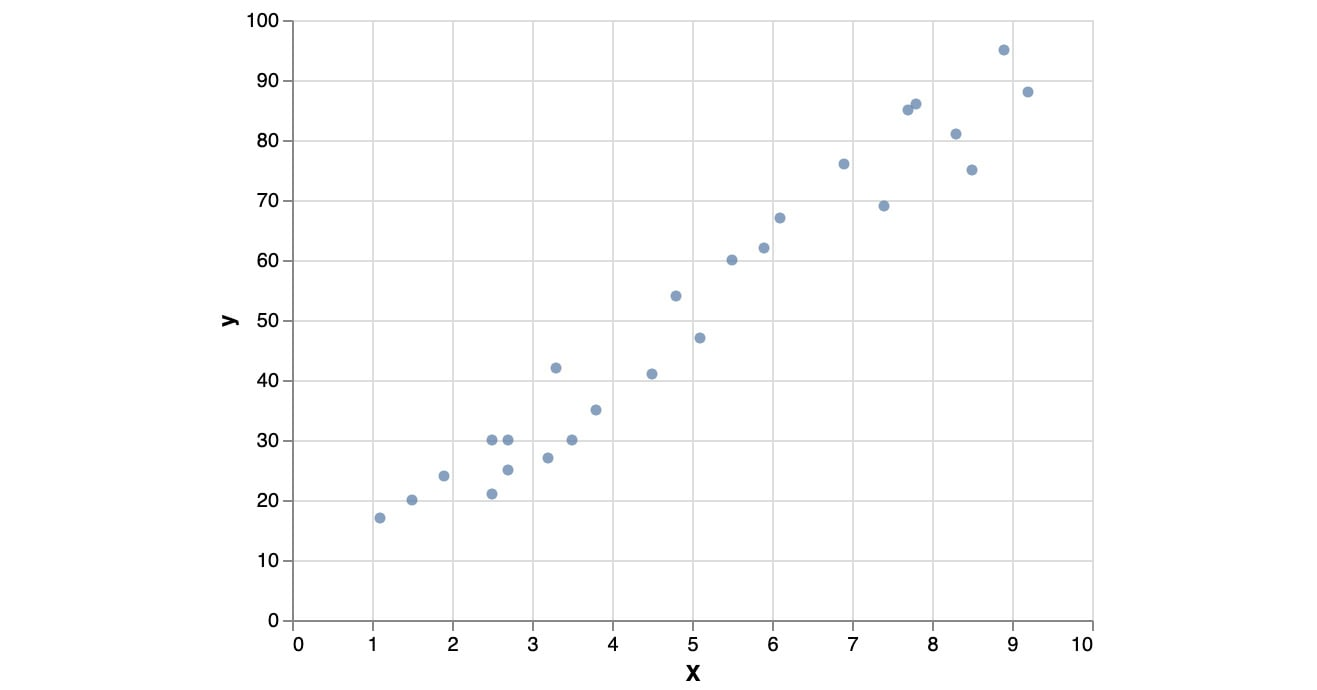Figure 1.39: Example of a dataset with one target variable and one feature

If we fit a linear regression on this dataset, the algorithm will try to find a solution for the following equation: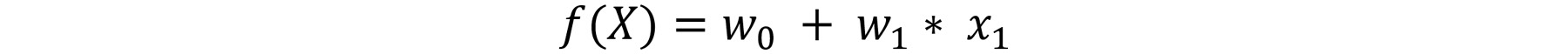Figure 1.40: Function f(x) for linear regression fitting on a dataset

So, it just needs to find the values of the `w``0` and `w`1 parameters that will approximate the data as closely as possible. In this case, the algorithm may come up with `w``o`` = 0` and `w``1`` = 10`. So, the function the model learns will be as follows: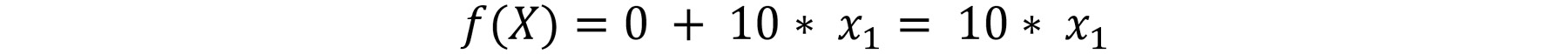Figure 1.41: Function f(x) using estimated values

We can visualize this on the same graph as for the data: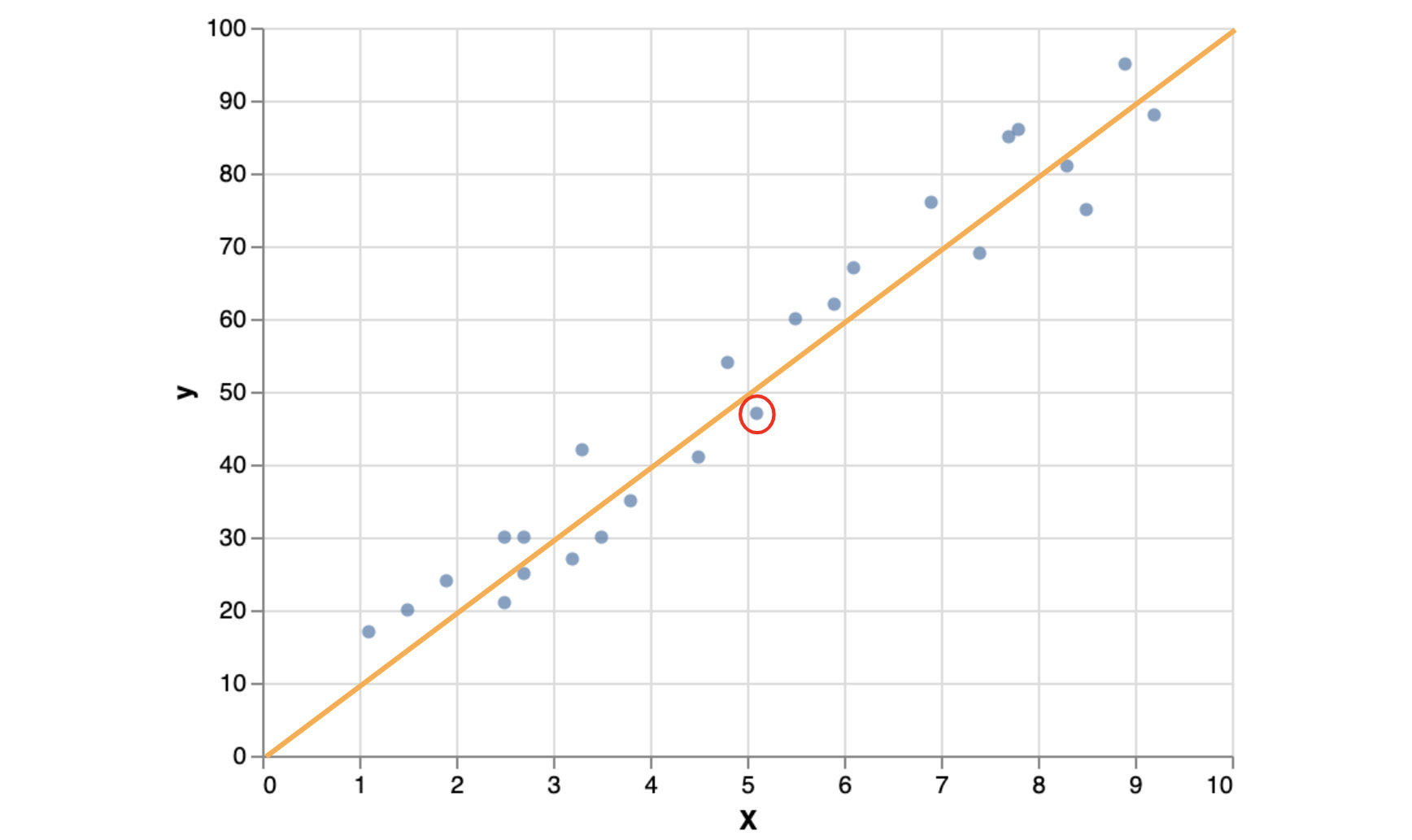Figure 1.42: Fitted linear model on the example dataset

We can see that the fitted model (the orange line) is approximating the original data quite closely. So, if we predict the outcome for a new data point, it will be very close to the true value. For example, if we take a point that is close to 5 (let's say its values are `x = 5.1` and `y = 48`), the model will predict the following: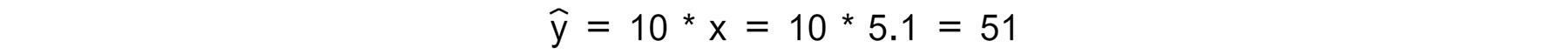Figure 1.43: Model prediction

This value is actually very close to the ground truth, 48 (red circle). So, our model prediction is quite accurate.

This is it. It is quite simple, right? In general, a dataset will have more than one feature, but the logic will be the same: the trained model will try to find the best parameters for each variable to get predictions as close as possible to the true values.

We just saw an example of linear models, but there are actually other types of machine learning algorithms, such as tree-based or neural networks, that can find more complex patterns from data.

### Model Hyperparameters

On top of the model parameters that are learned automatically by the algorithm (now you understand why we call it machine learning), there is also another type of parameter called the hyperparameter. Hyperparameters cannot be learned by the model. They are set by data scientists in order to define some specific conditions for the algorithm learning process. These hyperparameters are different for each family of algorithms and they can, for instance, help fast-track the learning process or limit the risk of overfitting. In this book, you will learn how to tune some of these machine learning hyperparameters.

### The sklearn API

As mentioned before, the scikit-learn (or `sklearn`) package has implemented an incredible amount of machine learning algorithms, such as logistic regression, k-nearest neighbors, k-means, and random forest.

Note

Do not worry about these terms—you are not expected to know what these algorithms involve just yet. You will see a simple random forest example in this chapter, but all of these algorithms will be explained in detail in later chapters of the book.

`sklearn` groups algorithms by family. For instance, `RandomForest` and `GradientBoosting` are part of the `ensemble` module. In order to make use of an algorithm, you will need to import it first like this:

`from sklearn.ensemble import RandomForestClassifier`

Another reason why `sklearn` is so popular is that all the algorithms follow the exact same API structure. So, once you have learned how to train one algorithm, it is extremely easy to train another one with very minimal code changes. With `sklearn`, there are four main steps to train a machine learning model:

1. Instantiate a model with specified hyperparameters: this will configure the machine learning model you want to train.
2. Train the model with training data: during this step, the model will learn the best parameters to get predictions as close as possible to the actual values of the target.
3. Predict the outcome from input data: using the learned parameter, the model will predict the outcome for new data.
4. Assess the performance of the model predictions: for checking whether the model learned the right patterns to get accurate predictions.

Note

In a real project, there might be more steps depending on the situation, but for simplicity, we will stick with these four for now. You will learn the remaining ones in the following chapters.

As mentioned before, each algorithm will have its own specific hyperparameters that can be tuned. To instantiate a model, you just need to create a new variable from the class you imported previously and specify the values of the hyperparameters. If you leave the hyperparameters blank, the model will use the default values specified by `sklearn`.

It is recommended to at least set the `random_state` hyperparameter in order to get reproducible results every time that you have to run the same code:

`rf_model = RandomForestClassifier(random_state=1)`

The second step is to train the model with some data. In this example, we will use a simple dataset that classifies 178 instances of Italian wines into 3 categories based on 13 features. This dataset is part of the few examples that `sklearn` provides within its API. We need to load the data first:

```from sklearn.datasets import load_wine

Then using the `.fit()` method to train the model, you will provide the features and the target variable as input:

`rf_model.fit(features, target)`

You should get the following output: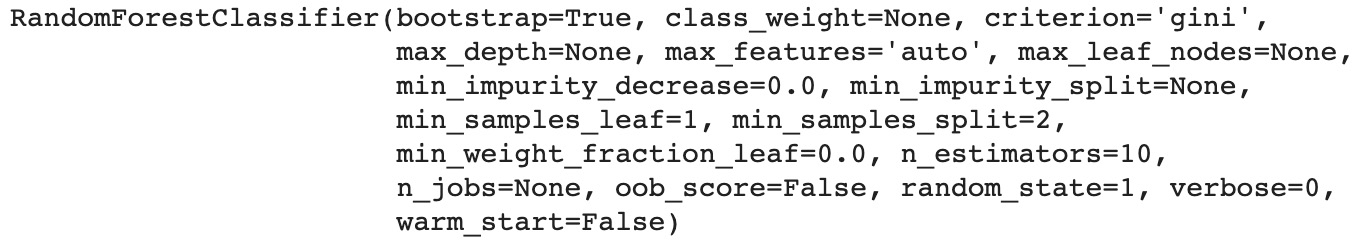Figure 1.44: Logs of the trained Random Forest model

In the preceding output, we can see a Random Forest model with the default hyperparameters. You will be introduced to some of them in Chapter 4, Multiclass Classification with RandomForest.

Once trained, we can use the `.predict()` method to predict the target for one or more observations. Here we will use the same data as for the training step:

```preds = rf_model.predict(features)
preds```

You should get the following output: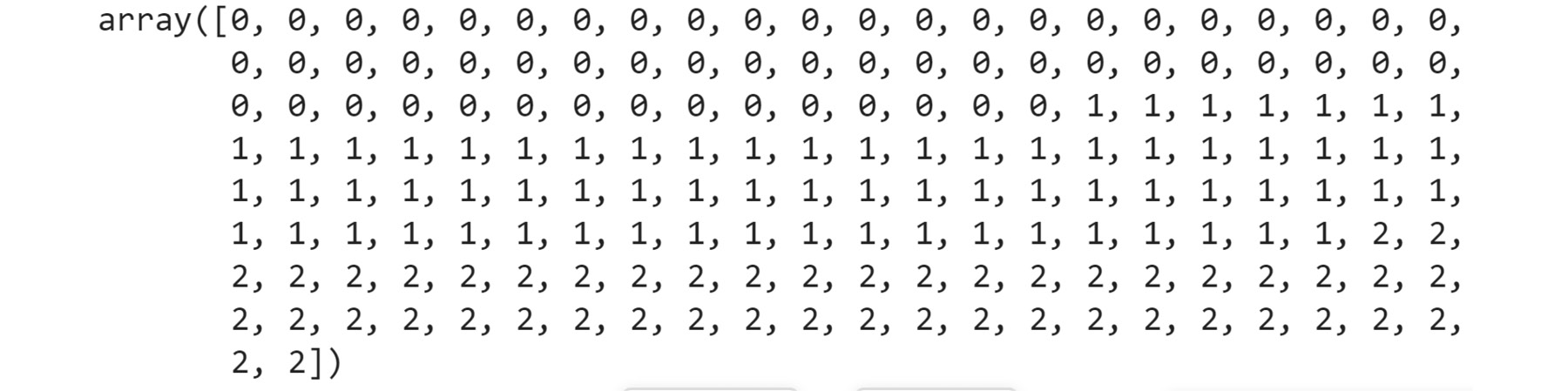Figure 1.45: Predictions of the trained Random Forest model

From the preceding output, you can see that the 178 different wines in the dataset have been classified into one of the three different wine categories. The first lot of wines have been classified as being in category 0, the second lot are category 1, and the last lot are category 2. At this point, we do not know what classes 0, 1, or 2 represent (in the context of the "type" of wine in each category), but finding this out would form part of the larger data science project.

Finally, we want to assess the model's performance by comparing its predictions to the actual values of the target variable. There are a lot of different metrics that can be used for assessing model performance, and you will learn more about them later in this book. For now, though, we will just use a metric called accuracy. This metric calculates the ratio of correct predictions to the total number of observations:

```from sklearn.metrics import accuracy_score
accuracy_score(target, preds)```

You should get the following output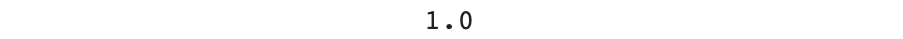Figure 1.46: Accuracy of the trained Random Forest model

In this example, the Random Forest model learned to predict correctly all the observations from this dataset; it achieves an accuracy score of `1` (that is, 100% of the predictions matched the actual true values).

It's as simple as that! This may be too good to be true. In the following chapters, you will learn how to check whether the trained models are able to accurately predict unseen or future data points or if they have only learned the specific patterns of this input data (also called overfitting).

## Exercise 1.03: Predicting Breast Cancer from a Dataset Using sklearn

In this exercise, we will build a machine learning classifier using `RandomForest` from `sklearn` to predict whether the breast cancer of a patient is malignant (harmful) or benign (not harmful).

The dataset we will use is the Breast Cancer Wisconsin (Diagnostic) dataset, which is available directly from the `sklearn` package at https://packt.live/2FcOTim.

1. Open a new Colab notebook.
2. Import the `load_breast_cancer` function from `sklearn.datasets`:
`from sklearn.datasets import load_breast_cancer`
3. Load the dataset from the `load_breast_cancer` function with the `return_X_y=True` parameter to return the features and response variable only:
`features, target = load_breast_cancer(return_X_y=True)`
4. Print the variable features:
`print(features)`

You should get the following output: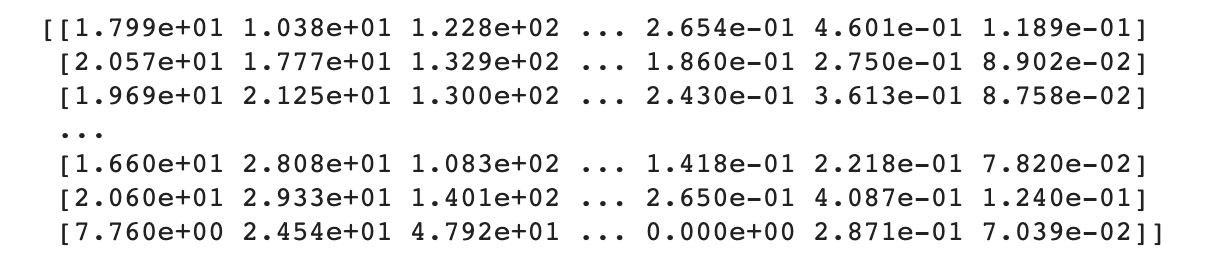Figure 1.47: Output of the variable features

5. Print the `target` variable:
`print(target)`

You should get the following output: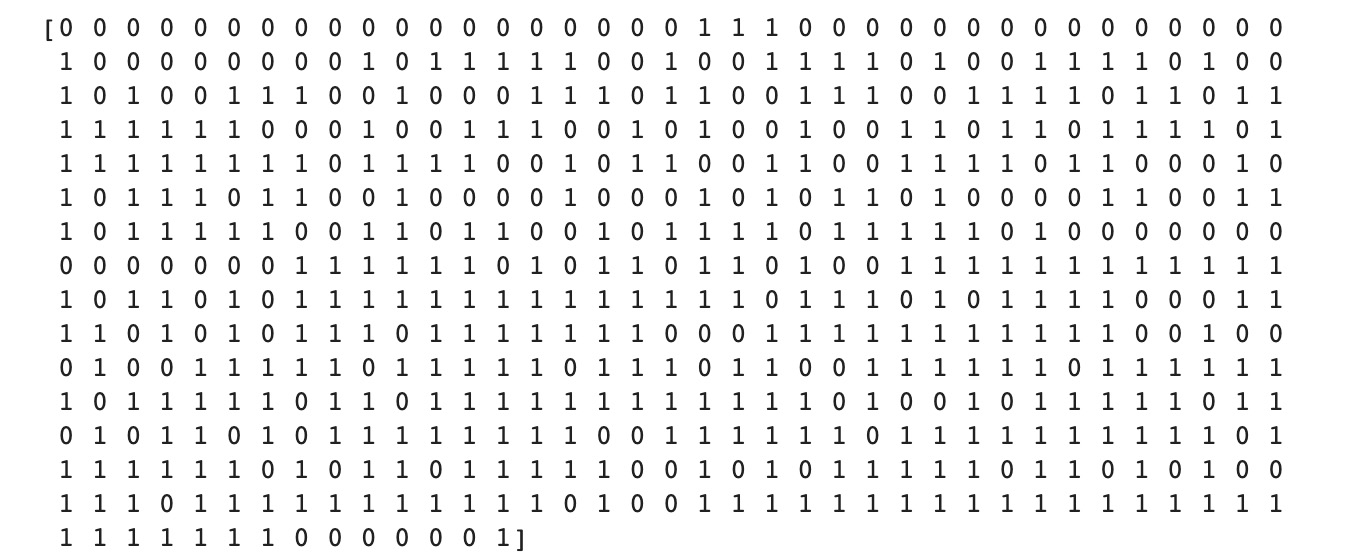Figure 1.48: Output of the variable target

The preceding output shows the values of the target variable. There are two classes shown for each instance in the dataset. These classes are `0` and `1`, representing whether the cancer is malignant or benign.

6. Import the `RandomForestClassifier` class from `sklearn.ensemble`:
`from sklearn.ensemble import RandomForestClassifier`
7. Create a new variable called `seed`, which will take the value `888` (chosen arbitrarily):
`seed = 888`
8. Instantiate `RandomForestClassifier` with the `random_state=seed` parameter and save it into a variable called `rf_model`:
`rf_model = RandomForestClassifier(random_state=seed)`
9. Train the model with the `.fit()` method with `features` and `target` as parameters:
`rf_model.fit(features, target)`

You should get the following output: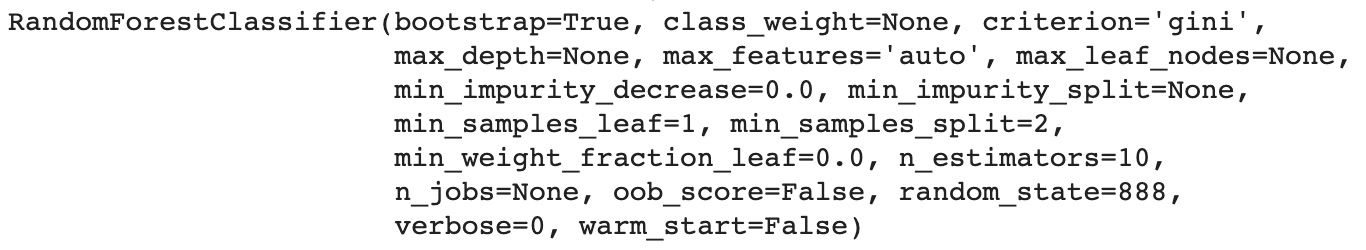Figure 1.49: Logs of RandomForestClassifier

10. Make predictions with the trained model using the `.predict()` method and `features` as a parameter and save the results into a variable called `preds`:
`preds = rf_model.predict(features)`
11. Print the `preds` variable:
`print(preds)`

You should get the following output: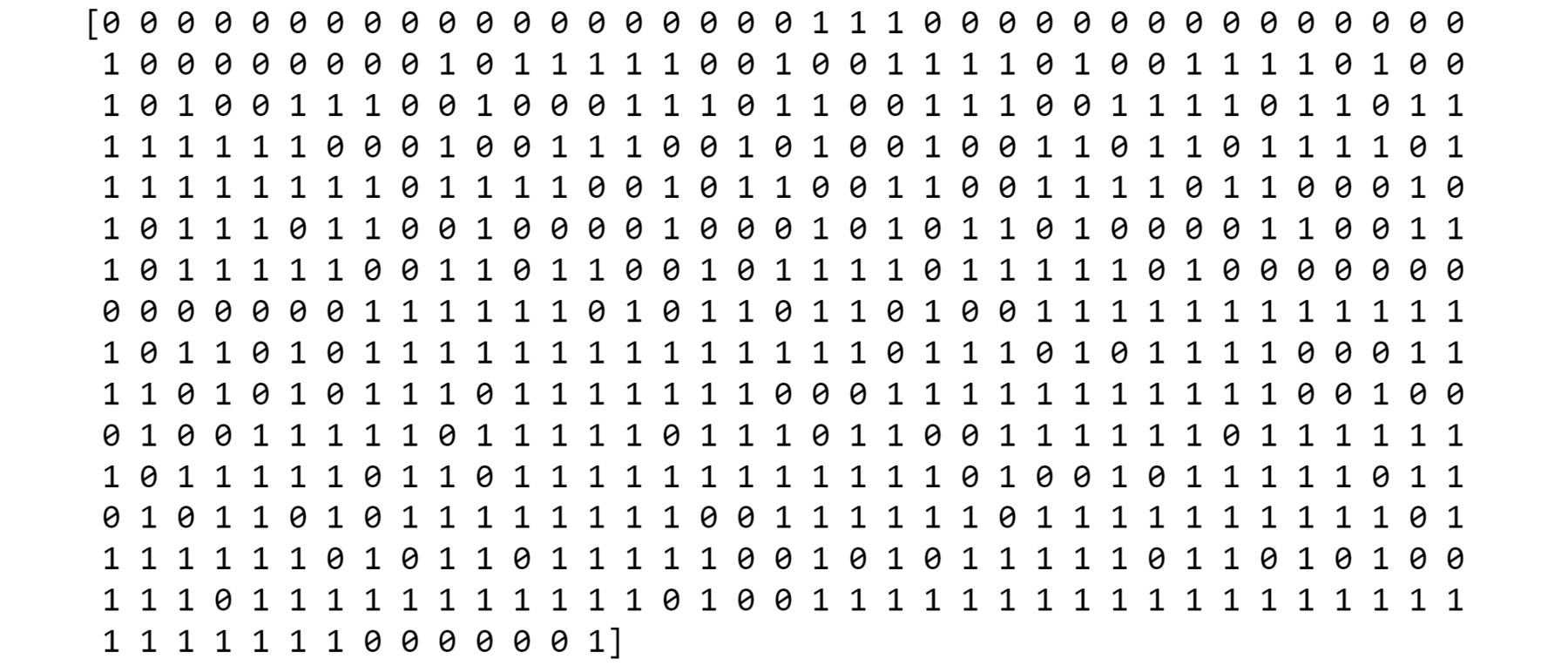Figure 1.50: Predictions of the Random Forest model

The preceding output shows the predictions for the training set. You can compare this with the actual target variable values shown in Figure 1.48.

12. Import the `accuracy_score` method from `sklearn.metrics`:
`from sklearn.metrics import accuracy_score`
13. Calculate `accuracy_score()` with `target` and `preds` as parameters:
`accuracy_score(target, preds)`

You should get the following output: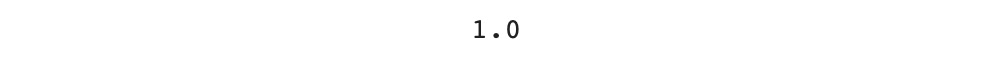Figure 1.51: Accuracy of the model

You just trained a Random Forest model using `sklearn` APIs and achieved an accuracy score of `1` in classifying breast cancer observations.

## Activity 1.01: Train a Spam Detector Algorithm

You are working for an email service provider and have been tasked with training an algorithm that recognizes whether an email is spam or not from a given dataset and checking its performance.

In this dataset, the authors have already created 57 different features based on some statistics for relevant keywords in order to classify whether an email is spam or not.

Note

The dataset was originally shared by Mark Hopkins, Erik Reeber, George Forman, and Jaap Suermondt: https://packt.live/35fdUUA.

1. Import the required libraries.
2. Load the dataset using `.pd.read_csv()`.
3. Extract the response variable using .`pop()` from `pandas`. This method will extract the column provided as a parameter from the DataFrame. You can then assign it a variable name, for example, `target = df.pop('class')`.
4. Instantiate `RandomForestClassifier`.
5. Train a Random Forest model to predict the outcome with .`fit()`.
6. Predict the outcomes from the input data using `.predict()`.
7. Calculate the accuracy score using `accuracy_score`.

The output will be similar to the following: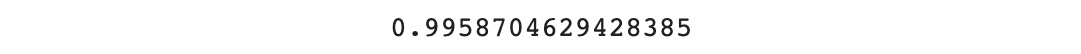Figure 1.52: Accuracy score for spam detector

Note

The solution to this activity can be found at the following address: https://packt.live/2GbJloz.

# Summary

This chapter provided you with an overview of what data science is in general. We also learned the different types of machine learning algorithms, including supervised and unsupervised, as well as regression and classification. We had a quick introduction to Python and how to manipulate the main data structures (lists and dictionaries) that will be used in this book.

Then we walked you through what a DataFrame is and how to create one by loading data from different file formats using the famous pandas package. Finally, we learned how to use the sklearn package to train a machine learning model and make predictions with it.

This was just a quick glimpse into the fascinating world of data science. In this book, you will learn much more and discover new techniques for handling data science projects from end to end.

The next chapter will show you how to perform a regression task on a real-world dataset.

• ##### Anthony So

Anthony So is a renowned leader in data science. He has extensive experience in solving complex business problems using advanced analytics and AI in different industries including financial services, media, and telecommunications. He is currently the chief data officer of one of the most innovative fintech start-ups. He is also the author of several best-selling books on data science, machine learning, and deep learning. He has won multiple prizes at several hackathon competitions, such as Unearthed, GovHack, and Pepper Money. Anthony holds two master's degrees, one in computer science and the other in data science and innovation.

Browse publications by this author
• ##### Thomas V. Joseph

Thomas V. Joseph is a data science practitioner, researcher, trainer, mentor, and writer with more than 19 years of experience. He has extensive experience in solving business problems using machine learning toolsets across multiple industry segments.

Browse publications by this author
• ##### Robert Thas John

Robert Thas John is a Google developer expert in machine learning. His day job involves working as a data engineer on the Google Cloud Platform by building, training, and deploying large-scale machine learning models. He also makes decisions about how to store and process large amounts of data. He has more than 10 years of experience in building enterprise-grade solutions and working with data. He spends his free time learning or contributing to the developer community. He frequently travels to speak at technology events or to mentor developers. He also writes a blog on data science.

Browse publications by this author
• ##### Andrew Worsley

Andrew David Worsley is an independent consultant and educator with expertise in the areas of machine learning, statistics, cloud computing, and artificial intelligence. He has practiced data science in several countries across a multitude of industries including retail, financial services, marketing, resources, and healthcare.

Browse publications by this author
• ##### Dr. Samuel Asare

Dr. Samuel Asare is a professional engineer with enthusiasm for Python programming, research, and writing. He is highly skilled in applying data science methods to the extraction of useful insights from large data sets. He possesses solid skills in project management processes. Samuel has previously held positions, in industry and academia, as a process engineer and a lecturer of materials science and engineering respectively. Presently, he is pursuing his passion for solving industry problems, using data science methods, and writing.

Browse publications by this author